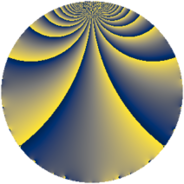# Properties

 Label 1089.1.hLevel $1089$ Weight $1$ Character orbit 1089.h Rep. character $\chi_{1089}(241,\cdot)$ Character field $\Q(\zeta_{6})$ Dimension $4$ Newform subspaces $1$ Sturm bound $132$ Trace bound $0$

# Related objects

## Defining parameters

 Level: $$N$$ $$=$$ $$1089 = 3^{2} \cdot 11^{2}$$ Weight: $$k$$ $$=$$ $$1$$ Character orbit: $$[\chi]$$ $$=$$ 1089.h (of order $$6$$ and degree $$2$$) Character conductor: $$\operatorname{cond}(\chi)$$ $$=$$ $$99$$ Character field: $$\Q(\zeta_{6})$$ Newform subspaces: $$1$$ Sturm bound: $$132$$ Trace bound: $$0$$

## Dimensions

The following table gives the dimensions of various subspaces of $$M_{1}(1089, [\chi])$$.

Total New Old
Modular forms 32 20 12
Cusp forms 8 4 4
Eisenstein series 24 16 8

The following table gives the dimensions of subspaces with specified projective image type.

$$D_n$$ $$A_4$$ $$S_4$$ $$A_5$$
Dimension 0 0 4 0

## Trace form

 $$4q + 2q^{3} + 2q^{4} + 2q^{5} - 2q^{9} + O(q^{10})$$ $$4q + 2q^{3} + 2q^{4} + 2q^{5} - 2q^{9} - 2q^{12} - 4q^{14} - 2q^{15} + 2q^{16} - 2q^{20} - 4q^{27} - 2q^{31} - 4q^{36} - 4q^{37} + 4q^{42} - 4q^{45} + 2q^{47} + 4q^{48} + 2q^{49} + 4q^{53} + 4q^{58} - 2q^{59} - 4q^{60} + 4q^{64} + 2q^{67} + 4q^{70} - 4q^{71} + 4q^{80} - 2q^{81} + 2q^{93} - 2q^{97} + O(q^{100})$$

## Decomposition of $$S_{1}^{\mathrm{new}}(1089, [\chi])$$ into newform subspaces

Label Dim. $$A$$ Field Image CM RM Traces $q$-expansion
$$a_2$$ $$a_3$$ $$a_5$$ $$a_7$$
1089.1.h.a $$4$$ $$0.543$$ $$\Q(\sqrt{-2}, \sqrt{-3})$$ $$S_{4}$$ None None $$0$$ $$2$$ $$2$$ $$0$$ $$q+(\beta _{1}-\beta _{3})q^{2}+(1-\beta _{2})q^{3}+(1-\beta _{2}+\cdots)q^{4}+\cdots$$

## Decomposition of $$S_{1}^{\mathrm{old}}(1089, [\chi])$$ into lower level spaces

$$S_{1}^{\mathrm{old}}(1089, [\chi]) \cong$$ $$S_{1}^{\mathrm{new}}(99, [\chi])$$$$^{\oplus 2}$$